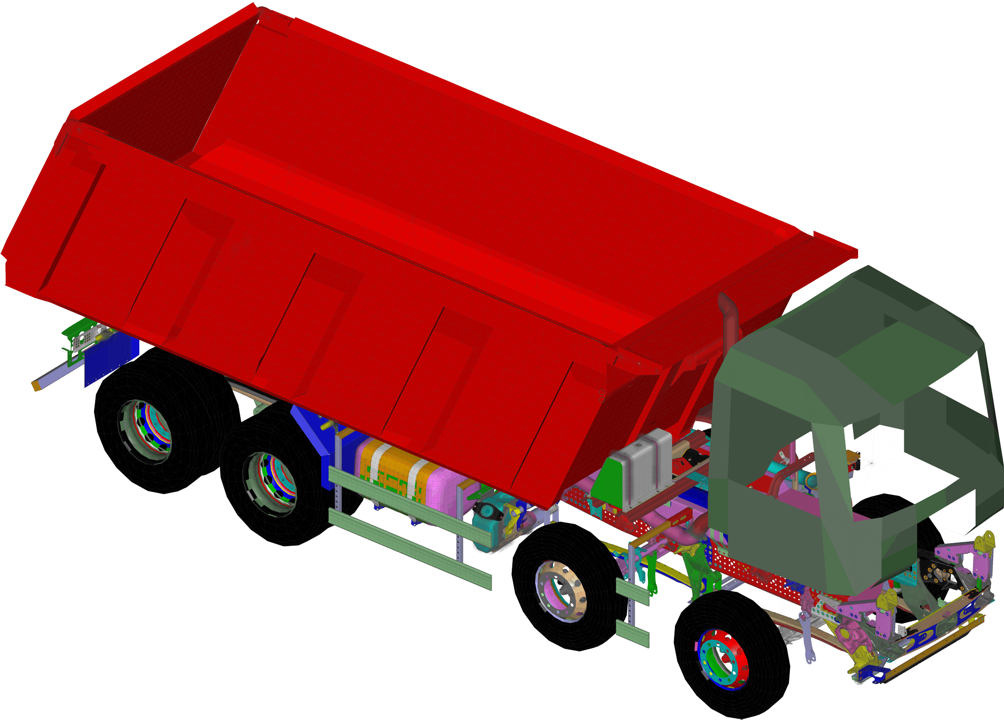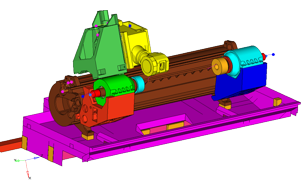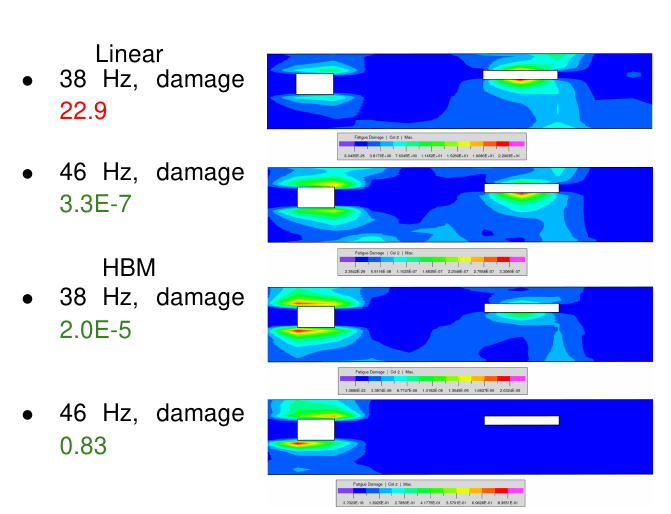# Dynamic Response

The Dynamic Response Analysis allows for the determination of structural responses in the time or frequency domain.

The solution of the dynamic equation is performed either directly using physical coordinates or in modal coordinates after a transformation into the modal space.

• The response in the time domain (transient response) is determined by an integration of the equation of motion:
• Absolute transient response with or without rigid body response.
• Direct integration of the equation of motion or integration after a transformation to the modal space. Available solvers are Newmark β; and HHT (Hilber-Hughes-Taylor)
Local nonlinear effects are taken into account by
• nonlinear spring elements,
• nonlinear damper elements, and
• nonlinear control elements.
• The response in the frequency domain (frequency response) is determined by the solution of the linear complex equation system for each excitation frequency requested:
• Absolute frequency response with or without rigid body response.
• Direct solution of the equation system or solution after a transformation to the modal space.
• Frequency and rotation speed dependent stiffness and damping can be taken into account with CONTROL6 element
• Without running through the transient phenomenon a calculation of the steady-state response can be performed. To achieve that, a number of frequency response analyses are superposed in the time domain. In addition, a static load case can be taken into account. This is facilitated for all periodic excitations with known harmonic composition.

By specification of a node set the run time and disk space for modal superposition methods can be drastically reduced when the results are determined for the set members only.
The features below hold for both time-history and frequency response methods:

Available damping features
• The damping properties may be modeled by one of the following methods:
• material or structural damping for elements,
• global structural damping for Components,
• proportional damping (Rayleigh damping),
• viscous damper elements,
• modal viscous damping,
• modal structural damping,
• modal structural damping,
• direct input of modal damping matrix.
• direct input of damping matrix.
For calculations in frequency domain, the structural damping may be defined as a function of frequency, alternatively. Frequency-dependent viscous damping parameters can be specified using a special element (i.e. CONTROL6 element).

• The excitation is defined by static loading cases modulated by functions of time respectively frequency. The load definitions may consist of:
• concentrated forces or moments,
• prescribed displacements.

Results
• The primary results are:
• displacements,
• velocities and
• accelerations.
For the drawing of xy-plots, these data may be output as a function of time respectively frequency.
• Moreover, the following results may be derived:
• reaction forces,
• stresses and stress resultants,
• strain energy,
• kinetic energy,
• specific sound radiation power density.

Additional functions for modal methods Static Mode Shapes can be generated in order to enhance the modal basis of dynamic modes. This has the following two advantages:
• In the low frequency range the results will become essentially more accurate.
• They allow to consider non-structural degrees of freedom like the internal state variables of control elements.

The static mode shapes can be specified using one of the following cases:

• Directly by nodal displacements,
• By results from another analysis,
• By natural loads of specified elements (like spring forces),
• Implicitly by internal degrees of freedom of controller elements.
Assembled situations can be used to highly accelerate frequency response analysis with many different load cases. Instead of solving all dynamic load cases separately, a combined response analysis can be performed.

Additional tools are available for the further processing of modal results:

• For the evaluation of a structural response modal participation factors of the primary results can be derived and exported.
• For the evaluation of the contribution of single degrees of freedom to a structural response node participation factors can be derived and exported.
• For a transient response a statistical evaluation of stresses or element forces over the time domain can be performed, which gives the maximal and effective values. These statistical values can be used in durability considerations, for example.Tipper truck, Daimler AGMachine tool, INDEX-Werke GmbH & Co. KG

## Harmonic Balance Method

The Harmonic Balance Method (HBM) is a steady state method to calculate solutions to nonlinear differential equations. In PERMAS it is used in the context of a nonlinear frequency response. A new class of applications is openend with this functionality, such as:
• Rattle noise,
• Squeak noise,
• Bolted joints and friction between parts,
• Vibro-impact problems,
• Lumped multi-degree-of-freedom (MDOF) and FE models with local nonlinearities.HBM+Fatigue

## Random Response Analysis

Frequently, vibrational loads are not predictable like for cars on a bumpy road, for a house under wind loads, or for a ship on rough sea.

Conveniently, such stochastic loads can be described by random processes. Correlations between such processes and their transformation into frequency domain are leading to the central concept of power spectral density.

One special phenomenon is white noise which describes a constant power spectral density over the full range of frequencies.

The loads are specified as power spectral densities and the results are derived as RMS quantities and power spectral densities as well.

This method is implemented as a modal method, i.e. an eigenvalue analysis is performed first followed by the response analysis in modal space and a subsequent back transformation into physical space where the results are made available for export and post-processing.

## Spectral Response Analysis

In case of a prescribed ground motion, like in earthquake analysis, the dynamic response behavior is determined by a special method, which results in maximum response values. There, the following requirements have to be fulfilled:
• uni-directional and translational motion of the ground,